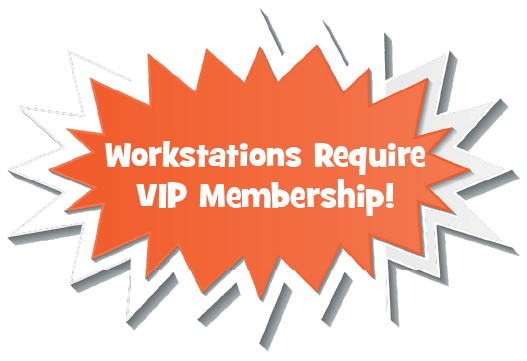## Multiplication Teaching Resources

Math workstations are a great way for students to engage in academically rigorous, engaging, standards based practice. In these workstations, students will work through a cycle of concrete, pictorial and abstract activities to practice their basic facts. There are artifact sheets so that there is evidence of student learning. There is a certificate at the end of each booklet. These activities can be used for workstations, in small guided math groups and for some homework.4,064 teaching resources that will make your classroom buzz

MULTIPLYING BY 2

MULTIPLYING BY 2

##### Multiplying By 3

MULTIPLYING BY 3

MULTIPLYING BY  3

MULTIPLYING BY 4

MULTIPLYING BY 4

##### Multiplying By 5

MULTIPLYING BY 5

MULTIPLYING BY 5

MULTIPLYING BY 6

MULTIPLYING BY 6

MULTIPLYING BY 7

MULTIPLYING BY 7

MULTIPLYING BY 8

MULTIPLYING BY 8

##### Multiplying By 9

MULTIPLYING BY 9

MULTIPLYING BY 9